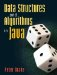# Analysis of Algorithms

One of the programming goals we mentioned in Chapter 1 was efficiency. In this chapter, we look at techniques for measuring efficiency. Section 7.1 shows how to directly time programs and points out some problems with this approach. Section 7.2 introduces some powerful mathematical tools to simplify our reasoning about the efficiency of algorithms. The connection between this math and actual algorithms is made in Section 7.3. Several variations on analysis, such as considering the average or the worst-case running time, are explored in Sections 7.4 and 7.5.

If the thought of doing math makes you nervous, you may wish to review Appendix C.Data Structures and Algorithms in Java
ISBN: 0131469142
EAN: 2147483647
Year: 2004
Pages: 216
Authors: Peter Drake